# The greatest common divisor and least common multiple

Definition: the Greatest common divisor of two or more natural numbers is called the greatest natural number that divides each of the given numbers.

For example

NOD## Coprime numbers

Definition: Two natural numbers are called relatively Primeif their GCD is equal to one.

## Finding GCD using the decomposition into Prime factors

### To find the GCD of two or more numbers, you must:

1. To put these numbers into Prime factors.
2. To make the product of the common Prime factors taken with the smallest exponent.
3. To find the value of the work.

### The Euclidean Algorithm

1. Share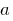onwith Stacey: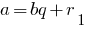2. Divide the divisorinto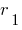:3. Divide the divisorinto the new balance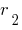: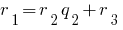The last nonzero remainder is the GCD.

## Least common multiple (LCM)

The least common multiple of two or more natural numbers is called the smallest natural number that is divisible by each of the given numbers.

## Finding knock two natural numbers

### To find the NOC two or more numbers, you must:

HCKFor example

NSK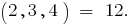## The connection between the NODE and the NOC of the two numbers

NOD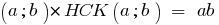Tags:
Chapter:
Versions in other languages:
Share with friends: